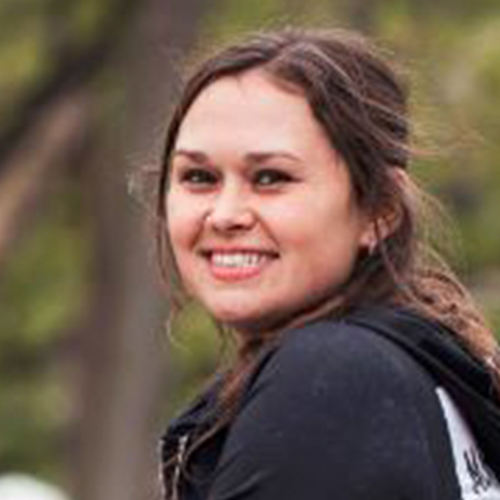• 3
Grade 3
• Number Properties
• 3.46
Compare Numbers Up to 1000
Taught by• 3.47
Put Numbers in Order Up to 120
Taught by• 3.48
Solve Inequalities
Taught by• 3.50
Value of Underlined Digit with Numbers Up to 1000
Taught by• 3.51
Convert Numbers with Tens, Hundreds, Thousands
Taught by• 3.52
Convert from Expanded Form
Taught by• 3.53
Convert Numbers with Hundreds, Thousands, Millions
Taught by• 3.111
Related Addition Equations Up to 10
Taught by• 3.112
Related Addition Equations Up to 1000
Taught by• 3.113
Related Subtraction Equations Up to 10
Taught by• 3.114
Related Subtraction Equations with Sums Up to 1000
Taught by• 3.115
Related Equations with Sums Up to 1000
Taught by• Counting
• 3.46
Compare Numbers Up to 1000
Taught by• 3.57
Count by a Specific Number
Taught by• 3.110
Counting and Number Patterns: Skip-Counting Sequences
Taught by• Comparison
• 3.47
Put Numbers in Order Up to 120
Taught by• 3.48
Solve Inequalities
Taught by• 3.98
Comparing Objects - Same / Different
Taught by• Mixed Equations
• 3.48
Solve Inequalities
Taught by• 3.115
Related Equations with Sums Up to 1000
Taught by• Addition
• 3.53
Convert Numbers with Hundreds, Thousands, Millions
Taught by• 3.58
Add Two Numbers Up to 1000
Taught by• 3.111
Related Addition Equations Up to 10
Taught by• 3.112
Related Addition Equations Up to 1000
Taught by• 3.117
Balance Addition Equations with Sums Up to 1000
Taught by• 3.118
Balance Addition Equations with Operands Up to 1000
Taught by• Money
• 3.54
Equivalent Coins I
Taught by• 3.55
Equivalent Coins II
Taught by• 3.56
Count Money
Taught by• 3.60
Price Lists
Taught by• 3.61
Consumer Math: Price Lists
Taught by• Number Patterns
• 3.57
Count by a Specific Number
Taught by• 3.107
Multiplication Input/Output Tables
Taught by• 3.109
Time Patterns
Taught by• 3.110
Counting and Number Patterns: Skip-Counting Sequences
Taught by• Subtraction
• 3.59
Subtract Two Numbers Up to 1000
Taught by• 3.113
Related Subtraction Equations Up to 10
Taught by• 3.114
Related Subtraction Equations with Sums Up to 1000
Taught by• 3.119
Balance Subtraction Equations with Operands Up to 1000
Taught by• Division
• 3.62
Division with Divisors Up to 10
Taught by• Multiplication
• 3.63
Multiply Two Numbers
Taught by• 3.64
Multiplication with a Specific Number Up to 12
Taught by• 3.107
Multiplication Input/Output Tables
Taught by• Measurement
• 3.65
Which Metric Unit of Length Is Appropriate?
Taught by• 3.77
Which Metric Unit Is Appropriate?
Taught by• 3.79
Compare and Convert Metric Units
Taught by• Time
• 3.66
Reading Clocks
Taught by• 3.67
Match Analog and Digital Clocks
Taught by• 3.68
Match Clocks and Time
Taught by• 3.69
Reading Clocks
Taught by• 3.80
Convert Time Units
Taught by• 3.109
Time Patterns
Taught by• Geometry
• 3.70
Find the Perimeter
Taught by• 3.71
Perimeter: Find the Missing Side Length
Taught by• 3.72
Area with Unit Squares and Units
Taught by• 3.73
Area with Unit Squares
Taught by• 3.74
Compare Area and Perimeter of Two Figures
Taught by• 3.75
Area of Rectangles
Taught by• 3.76
Area with Unit Squares
Taught by• 3.78
Volume of Cubes and Rectangular Prisms
Taught by• 3.81
Angles of 90, 180, 270 and 360 Degrees
Taught by• 3.82
Acute, Right, Obtuse, and Straight Angles
Taught by• 3.84
Which 2 Dimensional Shape Is Described?
Taught by• 3.86
Compare Sides and Corners
Taught by• 3.87
Count Edges
Taught by• 3.89
Count Faces
Taught by• 3.90
Count Edges, Vertices and Faces
Taught by• 3.91
Which Shape Is Described?
Taught by• 3.94
Classify Quadrilateral Shapes
Taught by• 3.95
Relate Planar and Solid Figures
Taught by• 3.96
Identify Reflections, Rotations and Translations
Taught by• 3.98
Comparing Objects - Same / Different
Taught by• 3.103
Symmetry
Taught by• Graphing
• 3.99
Coordinate Graphs with Decimals and Negative Numbers
Taught by• 3.100
Coordinate Graphs Review
Taught by• 3.101
Coordinate Graphs Review with Whole Numbers
Taught by• 3.102
Coordinate Graphs as Maps
Taught by• 3.120
Create Bar Graphs Using Tables
Taught by• 3.121
Create Bar Graphs
Taught by• 3.122
Create Line Plots
Taught by• 3.123
Create Line Plots II
Taught by• 3.124
Create Pictographs
Taught by• 3.125
Create Line Graphs
Taught by• 3.126
Interpret Bar Graphs
Taught by• 3.127
Interpret Line Plots
Taught by• 3.131
Convert Graphs to Input/Output Tables
Taught by• Stats
• 3.136
Calculate Mode
Taught by• 3.137
Calculate Mean, Median, Mode and Range
Taught by• 3.138
Calculate Mean
Taught by• 3.139
Making Predictions
Taught byThe third grade is the start of multiplication, mixed fractions, and more complex number properties. As the math skills start becoming more complicated, students may need some extra help to truly grasp the concepts. Our teachers help fill the gaps in learning and offer their best tips in figuring out

• Students learn about angles, multiplication rules, measurements, and other third-grade math skills.
• On-demand videos for math skills by teachers who specialize in teaching math to younger students.
• Learn and master the fundamentals of algebra to prepare for more complex concepts in the next grade.